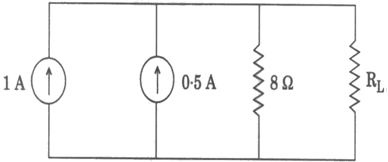Thursday 04th June 2020
 CBSE Guess > Papers > Question Papers > Class XII > 2010 > Electrical Engineering Electrical Engineering 2010 Question Paper For CBSE Class XII Exams (a) Explain with diagrams what do you understand by (3 Marks) (i) In phase (ii) Lagging (iii) LeadingAs applied to sinusoidal quantities. OR (b) A coil when connected to D.C supply of 100 volt takes 10 Ampere. When it is connected to A.C supply of 100 volt, 50 Hz it takes 5 Ampere. Calculate Resistance and Inductance of the coil.(4 Marks)OR(a) State Thevenin's Theorem and explain it with the help of simple network. (5 Marks) (b) What is resonance? (2 Marks) (a) Explain the significance of power factor in an A.C. circuit. (3 Marks) Calculate the value of load resistance R_L for maximum power transformation inthe circuit shown below. Also calculate the value of maximum power. (2+2 Marks) (b) Describe in brief the working of a manual Voltage Stabiliser with its circuit diagram. (5 Marks)What are the methods of measurement of power in a 3- phase system? Explain two wattmeter methods. (2+4 Marks) (a) Deduce the e.m.f. equation of a transformer. (5 Marks) (b) Write merits and demerits of ordinary transformer and auto - transformer. (5 Marks) (a) What is the principle of generation and motoring? Explain with block diagrams. (6 Marks) Explain with neat sketches the functions of each part of a D.C machine.Write the relationship between terminal voltage and induced e.m.f. for motoring and generating action. (6+4 Marks) Name various types of 1-phase motors. (b) Explain the construction and principle of shaded pole motor. (7 Marks) What are the advantages and disadvantages of 3- phase induction motors over 1-phase induction motors? (4 Marks) Electrical Engineering 2010 Question Papers Class XII Delhi Foreign HTML Format N/A PDF N/A CBSE 2010 Question Papers Class XII